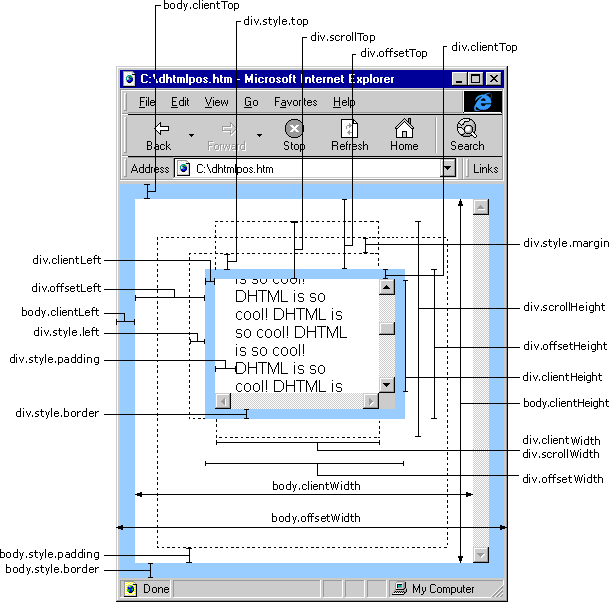# node

Node 模块提供了对DOM节点的最高层的封装，可以创建、操作、遍历、查找Dom节点。封装后的Node节点在各个浏览器端均具有一致的API，这些API甚至在NodeList类型上也是可以用的。同时，Node还提供了对CSS、动画、事件的基本的封装。可以让你方便的操作DOM节点。

## 载入Node模块

``````// 载入 Node 模块
KISSY.use('node',function(S,Node){
// 装载了 Node 模块，并处于可用状态
});``````

## 使用Node

Node API 是基于DOM Api 实现的，定义了一系列的语法糖，让用户更舒服的使用Node api，写的代码更加优雅。如果你对标准jQuery DOM API很了解，那么你对Node API也会非常熟悉。

## 查找节点

``````// 使用 S.one( [css selector string] )
var node = S.one('#main');

// 或者传入一个HtmlElement元素
var bodyNode = S.one(document.body);``````

`one()`方法类似于jquery的\$，通过传入选择器或者Dom实例来获取目标（包装后）的Node节点。如果传入一个css选择器，`one()`函数将返回第一个匹配的节点。如果要获得节点列表，需要使用`all()`方法。

KISSY 会根据浏览器平台加载正确的代码，用户不用关心平台，KISSY 一定会调用最合适、性能最优的选择器实现。在1.4.1中，不需要用户手动引用`sizzle`模块了，KISSY 会自行判断加载。

## 操作内容

KISSY Node 节点支持链式调用:

``````S.one('#test')
.parent('.fathor')
.next()
.html('<p></p>')
.on('click', function() { /* ... */ });``````

``S.Node('<div>hello kissy</div>').appendTo('body');``

## 访问 Node 节点的属性

``````var imgNode = S.one('#preview');
var bigSrc = imgNode.attr('src');//得到imgNode的src属性

imgNode.attr('src', 'new.png');// 设置src属性为一个新的值
imgNode.next().html('hello world');// 设置imgNode下一个兄弟节点的innerHTML``````

Node 实例通过`attr`方法来读写常见的属性。一些className和innerHTML相关的常用操作，被封装为addClass、replaceClass或者html方法。更多用法请参照下文API部分。

## Node 事件操作

``````S.one('#demo').on('click', function(e) {
e.halt();
alert('event: ' + e.type + ' target: ' + e.target.tagName);
});``````

## NodeList 集合

``````var doneTasks = S.all('#tasklist .completed');

// 针对所有的节点进行操作

// 调用each()方法来遍历这些节点
});``````

Node 实例支持逐级查找`S.all('.s1').all('.s2')``S.all('.s1 .s2')`是等价的。

`S.all('.s1').all('.s2')``S.all('.s1 .s2')`毕竟还是不同的，在一个多次遍历的场景中，无报错的情况下，通常第一个用法速度优于第二个，因为S.one始终从document根节点开始查找。

KISSY 支持几乎所有的CSS3选择器。CSS3选择器种类如下：

## 无障碍支持

KISSY 支持标准的ARIA。即 KISSY 可以完整的支持无障碍特性。比如对rolesstate的支持。这些特性可以和读屏软件很好的兼容，在增强html标签语义化的同时，让盲人用户使用页面更加顺畅。

``````// 写一个属性
var node = S.one('#toolbar').attr('role', 'toolbar');
// 同时写多个属性
node.attr({
});``````

# Node或NodeList 的实例方法

### all()，one()

``````S.one('.filter'); // 从document根节点开始查找一个节点
S.all('.filter'); // 从document根节点开始查找一批节点
node.one('.child'); // 得到当前节点下的匹配选择器的一个节点
node.all('.children'); // 得到当前节点下的匹配选择器的节点列表``````

### getDOMNode()，getDOMNodes()

``````node.getDOMNode();// 得到一个原生节点
node.getDOMNodes();// 得到一个原生节点组成的数组``````

### end()

``````S.one("body").all(".d1").css("color", "red");// => 返回节点.d1
S.one("body").all(".d1").css("color", "red").end();// => 返回节点 body
S.one("body").all(".d1").css("color", "red")
.end()
.all('.d2');// => 返回节点 .d2 ``````

### equals()

``````<div class='a b' id='a'></div>
<div class='a b' id='b'></div>
<script>
KISSY.all(".a").equals(KISSY.all(".b")) // => true
</script>``````

``````<p>1</p><div>2</div>
<script>
var pdiv = S.all("p");
var all= pdiv.add("div");  //  pdiv 不会变化
all.css("color","red"); // => 1,2 都为红字
</script>``````

### item()

``````<div class='a' id='a'></div>
<div class='a' id='b'></div>
<script>
// 取第0个节点
S.all(".a").item(0).attr("id")  // => a
// 取第一个节点
S.all(".a").item(1).attr("id")  // => b
// 取第二个节点
S.all(".a").item(2)  // => null
</script>``````

### slice()

``````var as = S.all(".a");
var subs = as.slice(1,3); // => subs != as
subs.length // => 2，subs 为被截取之后的节点 ``````

### scrollTop()

``````var p = S.all("p:first").scrollTop();

``S.one('.filter').scrollTop(100);// 设置元素.filter的滚动条高度为100``

``````KISSY.one(document).scrollTop();
KISSY.DOM.scrollTop();// 这两者完全一致``````

### height() 和 width()``````S.all(window).height(); // 得到浏览器可以区域的高度, 相当于 DOM.viewportHeight()
S.all(document).height(); //得到 html 文档的高度, 相当于 DOM.docHeight()``````

``````S.all('.filter').height(100);// 设置一组元素的高度为100px
S.all('.filter').height('100px');// 设置一组元素的高度为100px
S.all('.filter').height('20em');// 设置一组元素的高度为20em````````node.addStyleSheet('xx {_width:xx;}');// 可以写css hack``

``var styleEl = S.all("<style>p {color:red}</style>").appendTo("head");``

### append()

``````<h2>Greetings</h2>
<div class="container">
<div class="inner">Hello</div>
<div class="inner">Goodbye</div>
</div>
<script>
KISSY.use("node",function(S,Node){
Node.all('.inner').append('<p>Test</p>');
});
</script>``````

``````<h2>Greetings</h2>
<div class="container">
<div class="inner">
Hello
<p>Test</p>
</div>
<div class="inner">
Goodbye
<p>Test</p>
</div>
</div>``````

``S.all('.container').append(S.all('h2'));``

### appendTo()

``S.all('<p>Test</p>').appendTo('.inner');``

``S.all('h2').appendTo(S.all('.container'));``

### insertBefore()

``S.all('<p>Test</p>').insertBefore('.inner');``

``S.all('h2').insertBefore(S.all('.container'));``

### animate()

``````var node=KISSY.all(".foo");
node.animate({
width:100,
height:300
}, {
duration: 2000,
easing:'easeBoth' ,
complete: function () {
}
});``````

``````KISSY.all(".foo").each(function(n){
new KISSY.Anim(n,...).run();
});``````

### show()

``````node.show();// 直接显示
node.show(0.5,function(){
// 0.5 秒后执行这里的回调
});``````

### hide()

``````node.hide();// 直接隐藏
node.hide(0.5,function(){
// 0.5 秒后执行这里的回调
});``````

### toggle()

``````node.toggle();// 直接切换显示状态
node.toggle(0.5,function(){
// 0.5 秒后执行这里的回调
});``````

``````node.fadeIn(1,function(){
// 1 秒后执行回调
},easeIng);
// easyIng 取值请参照Anim``````

### filter()

``````KISSY.use('node',function(S,Node)){
var els = S.all('a').filter('.container');
// 过滤出className为container的a标签
});``````

### test()

``````var els = S.all('a');
console.log(els.test('.container'));//a标签是否都包含class名container``````

### clone()

• deep:false
• withDataAndEvent:false
• deepWithDataAndEvent:false

``````<div class="container">
<div class="hello">Hello</div>
<div class="goodbye">Goodbye</div>
</div>``````

``KISSY.one('.hello').appendTo('.goodbye');``

DOM 结构变为：

``````<div class="container">
<div class="goodbye">
Goodbye
<div class="hello">Hello</div>
</div>
</div>``````

``KISSY.one('.hello').clone(true).appendTo('.goodbye');``

``````<div class="container">
<div class="hello">Hello</div>
<div class="goodbye">
Goodbye
<div class="hello">Hello</div>
<div class="hello">Hello</div>
</div>
</div>``````

``node.clone(true,true);``

``node.clone(true,true,true);``

``````var elem=KISSY.one(".hello").attr("custom",{x:1});
elem.clone().attr("custom",{x:2});``````

### replaceClass()

className的替换

``````// 将.container的className类名更改为other-class
S.one('.container').replaceClass('container','other-class');``````

### attr()

``````// 批量设置属性
node.attr({
{ src: 'kissy.png', width: 400, height: 400 }
});
node.attr('id','myId');// 设置单个属性
node.attr('id');//=>得到id的值``````

### prop()

``````node.attr('data-my-key','my-value');// 有效
node.prop('data-my-key','my-value');// 无效``````

``````var c = S.Node("<input type='checkbox' checked='checked'/>");
console.log(c.attr('checked'));//=>'checked'
console.log(c.prop('checked'));//=>true
console.log(c.attr('nodeName'));//=> undefined
console.log(c.prop('nodeName').toLowerCase());// => input

c.prop("disabled", false); // ok
c.prop("checked", true); // ok
c.val("someValue"); // ok``````

Demo:selectionStart/End 兼容

### val()

``````node.val(); // 读
node.val('test');// 写``````

### css()

``````// 写单个样式
node.css('position','absolute');
// 批量写样式
node.css({position: 'absolute', top: '10px', left: '10px'});
// 读样式
node.css('position');``````

### offset()

``````// 读位置
var a = node.offset();
/* a 是一个坐标对象
{
top:100,
left:20
}
*/

// 写位置
node.offset({
'top':100,
'left':20
});``````

### scrollIntoView()

``````node.scrollIntoView();// 将node显示在视口范围内
node.scrollIntoView(true);// 强制上端和左端与窗口上端和左端对齐
//第二个参数：是否允许容器左右滚动以保证元素显示在其可视区域.
node.scrollIntoView(true,true);``````

### parent()

``````node.parent();//返回父元素
node.parent('.container');//返回满足选择器格式的父级元素，会一直向上找，直到document
node.parent(['.a','.b']);//同时查找多个
// 可以通过自定义函数来查找父级节点
node.parent(function(p){
// 注意：这里p是原生节点
return p.className == 'myclass';
});``````

### index()

``````node.index();// => 返回本节点在兄弟节点中的索引位置
node.index('selector');// => 返回node在匹配选择器节点中的位置``````

### next()

``````node.next();//下一个兄弟节点，若无，返回null
node.next('.class');//返回下一个符合选择器的兄弟节点``````

### first()

``````node.first();//返回node第一个子节点
nodelist.first();//返回nodelist里第一个node的第一个子节点``````

### siblings()

``````node.siblings();//返回node所有兄弟节点
node.siblings('.filter');//获得符合‘.filter’的兄弟节点``````

### children()

``````node.children();//返回所有子节点
node.children('.filter');返回符合选择器的所有子节点``````

### contains()

``````node.contains('.selector');// 是否包含选择器匹配的（第一个）节点
node.contains(otherNode);//传入node参数
node.contains([node1,node2]);//传入数组
node.contains(domNode);//传入裸的节点亦可
node.contains(nodeList);//这时会判断nodeList的第一个元素是否和node具有包含关系``````

### html()

``````node.html();//返回innerHTML
node.html('abc');//设置node的innerHTML

### data()

``````node.data('data-size','100');// data-size 的 expando , 值为 100;
node.data('data-size');//100``````

``````// 给dom元素写了一个伪属性
S.one('button').attr('data-x','asd');
// 通过data 是读不出来的
console.log(S.one('button').data('data-x'));``````

``````// 给dom元素写了一个扩展属性expando
S.one('button').data('data-x','asd');
// 通过data 是可以读出来的，但无法通过浏览器工具查看到button上有这个属性data-x
console.log(S.one('button').data('data-x'));``````

### innerWidth()

``````<div style="width: 100px;">
<div id="test" style="width: 80%; height: 20px; padding: 10px;"></div>
</div>
<script>
var S = KISSY, DOM = S.DOM,
elem = S.get('#test');

DOM.innerWidth(elem); // 返回 100
DOM.innerHeight(elem); // 返回 40
</script>``````

### outerWidth()

``````<div style="width: 100px;">
<div id="test" style="width: 80%;
height: 20px;
border: 2px solid gray;
margin: 6px;"></div>
</div>
<script>
var S = KISSY, DOM = S.DOM,
elem = S.get('#test');

DOM.outerWidth(elem); // 返回 104
DOM.outerHeight(elem); // 返回 44
DOM.outerWidth(elem, true); // 返回 116
DOM.outerHeight(elem, true); // 返回 56
</script>``````

### on()

on 方法是给文档添加行为的主要方式. 所有的事件类型, 例如 focus , mouseover , resize 都是有效的事件类型。window 的 beforeunload 和 error 事件使用了不标准的方式, 该方法不支持, 请直接在 window 对象上注册事件处理器.

``````S.one('#foo').on('click',function(){
// 其中this是原生节点
});``````

### fire()

``````node.on('click',function(){
// 其中this是原生节点